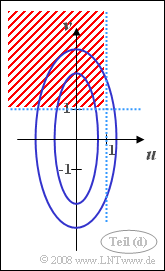# Exercise 4.4: Two-dimensional Gaussian PDF

We consider two-dimensional random variables,  where both components are always assumed to be mean-free.

• The 2D–PDF of the random variable  $(u, v)$  is:
$$f_{uv}(u, v)={1}/{\pi} \cdot {\rm e}^{-(2u^{\rm 2} \hspace{0.05cm}+ \hspace{0.05cm}v^{\rm 2}\hspace{-0.05cm}/\rm 2)}.$$
• The following parameters are known from another two-dimensional random variable  $(x, y)$  which is also Gaussian:
$$\sigma_x= 0.5, \hspace{0.5cm}\sigma_y = 1,\hspace{0.5cm}\rho_{xy} = 1.$$
• The values of the Gaussian error integral  ${\rm \phi}(x)$  and the complementary function  ${\rm Q}(x) = 1- {\rm \phi}(x)$  can be found in the adjacent table.

Hints:

Part 1:   Gaussian random variables without statistical bindings,
Part 2:   Gaussian random variables with statistical bindings.

### Questions

1

Which of the statements are true with respect to the two-dimensional random variable  $(u, v)$ ?

 The random variables  $u$  and  $v$  are uncorrelated. The random variables  $u$  and  $v$  are statistically independent.

2

Calculate the two standard deviations  $\sigma_u$  and  $\sigma_v$.  Enter the quotient of the two standard deviations as a check.

 $\sigma_u/\sigma_v \ = \$

3

Calculate the probability that  $u$  is less than  $1$ .

 ${\rm Pr}(u < 1)\ = \$

4

Calculate the probability that the random variable  $u$  is less than  $1$  and at the same time the random variable  $v$  is greater than  $1$  .

 ${\rm Pr}\big[(u < 1) ∩ (υ > 1)\big]\ = \$

5

Which of the statements are true for the two-dimensional random variable  $(x, y)$?

 The joint PDF $f_{xy}(x, y)$  is always zero outside the straight line  $y = 2x$. For all pairs of values on the straight line  $y = 2x$  holds:  $f_{xy}(x, y)= 0.5$. With respect to the edge PDFs:  $f_{x}(x) = f_{u}(u)$  and $f_{y}(y) = f_{v}(v)$ holds.

6

Calculate the probability that  $x$  is less than  $1$.

 ${\rm Pr}(x < 1)\ = \$

7

Now calculate the probability that the random variable  $x$  is less than  $1$  and at the same time the random variable  $y$  is greater than  $1$.

 ${\rm Pr}\big[(x < 1) ∩ (y > 1)\big]\ = \$

### Solution

#### Solution

(1)  Both statements are true:

• Comparing the given 2D–PDF with the general 2D–PDF
$$f_{uv}(u,v) = \frac{\rm 1}{{\rm 2}\it\pi \cdot \sigma_u \cdot \sigma_v \cdot \sqrt{{\rm 1}-\it \rho_{\it uv}^{\rm 2}}} \cdot \rm exp\left[\frac{\rm 1}{2\cdot (\rm 1-\it \rho_{uv}^{\rm 2}{\rm )}}(\frac{\it u^{\rm 2}}{\it\sigma_u^{\rm 2}} + \frac{\it v^{\rm 2}}{\it\sigma_v^{\rm 2}} - \rm 2\it\rho_{uv}\frac{\it u\cdot \it v}{\sigma_u\cdot \sigma_v}\rm )\right],$$
so it can be seen that no term with  $u \cdot v$  occurs in the exponent,  which is only possible with  $\rho_{uv} = 0$.
• But this means that  $u$  and  $v$  are uncorrelated.
• For Gaussian random variables,  however,  statistical independence always follows from uncorrelatedness.

(2)  With statistical independence holds:

$$f_{uv}(u, v) = f_u(u)\cdot f_v(v)$$
$$f_u(u)=\frac{{\rm e}^{-{\it u^{\rm 2}}/{(2\sigma_u^{\rm 2})}}}{\sqrt{\rm 2\pi}\cdot\sigma_u} ,$$
$$\it f_v{\rm (}v{\rm )}=\frac{{\rm e}^{-{\it v^{\rm 2}}/{{\rm (}{\rm 2}\sigma_v^{\rm 2}{\rm )}}}}{\sqrt{\rm 2\pi}\cdot\sigma_v}.$$
• By comparing coefficients,  we get  $\sigma_u = 0.5$  and  $\sigma_v = 1$.
• The quotient is thus  $\sigma_u/\sigma_v\hspace{0.15cm}\underline{=0.5}$.

(3)  Because  $u$  is a continuous random variable:

$$\rm Pr(\it u < \rm 1) = \rm Pr(\it u \le \rm 1) =\it F_u\rm (1).$$
• With the mean  $m_u = 0$  and the standard deviation  $\sigma_u = 0.5$  we get:
$$\rm Pr(\it u < \rm 1) = \rm \phi({\rm 1}/{\it\sigma_u})= \rm \phi(\rm 2) \hspace{0.15cm}\underline{=\rm 0.9772}.$$$\rm Pr\big[(\it u < \rm 1) \cap (\it v > \rm 1)\big]$

(4)  Due to the statistical independence between  $u$  and  $v$  holds:

$$\rm Pr\big[(\it u < \rm 1) \cap (\it v > \rm 1)\big] = \rm Pr(\it u < \rm 1)\cdot \rm Pr(\it v > \rm 1).$$
• The probability  ${\rm Pr}(u < 1) =0.9772$  has already been calculated.
• For the second probability  ${\rm Pr}(v > 1)$  holds for reasons of symmetry:
$$\rm Pr(\it v > \rm 1) = \rm Pr(\it v \le \rm (-1) = \it F_v\rm (-1) = \rm \phi(\frac{\rm -1}{\it\sigma_v}) = \rm Q(1) =0.1587$$
$$\Rightarrow \hspace{0.3cm} \rm Pr\big[(\it u < \rm 1) \cap (\it v > \rm 1)\big] = \rm 0.9772\cdot \rm 0.1587 \hspace{0.15cm}\underline{ = \rm 0.1551}.$$

The sketch on the right illustrates the given constellation:

• The PDF contour lines  (blue)  are stretched ellipses due to  $\sigma_v > \sigma_u$  in vertical direction.
• Drawn in red  (shading)  is the area whose probability should be calculated in this subtask.

(5)  Correct are  the first and the third suggested solutions:

• Because  $\rho_{xy} = 1$  there is a deterministic correlation between  $x$  and  $y$
⇒   All values lie on the straight line  $y =K \cdot x$.
• Because of the standard deviations  $\sigma_x = 0.5$  and  $\sigma_y = 1$  it holds  $K = 2$.
• On this straight line  $y = 2x$   ⇒   all PDF values are infinitely large.
• This means:   The joint PDF is here a  "Dirac wall".
• As you can see from the sketch,  the PDF values are Gaussian distributed on the straight line $y = 2x$.
• The two marginal probability densities are also Gaussian functions,  each with zero mean.
• Because of  $\sigma_x = \sigma_u$  and  $\sigma_y = \sigma_v$  also holds:
$$f_x(x) = f_u(u), \hspace{0.5cm}f_y(y) = f_v(v).$$

(6)  Since the PDF of the random variable  $x$  is identical to the PDF  $f_u(u)$,  it also results in exactly the same probability as calculated in the subtask  (3):

$$\rm Pr(\it x < \rm 1) \hspace{0.15cm}\underline{ = \rm 0.9772}.$$

(7)  The random event  $y > 1$  is identical to the event  $x > 0.5$.

• Thus, the wanted probability is equal to:
$${\rm Pr}\big[(x < 1) ∩ (y > 1)\big] = \rm Pr \big[ (\it x < \rm 1)\cap (\it x > \rm 0.5) \big] = \it F_x \rm( 1) - \it F_x\rm (0.5).$$
• With the standard deviation  $\sigma_x = 0.5$  it follows further:
$$\rm Pr \big[(\it x > \rm 0.5) \cap (\it x < \rm 1)\big] = \rm \phi(\rm 2) - \phi(1)=\rm 0.9772- \rm 0.8413\hspace{0.15cm}\underline{=\rm 0.1359}.$$